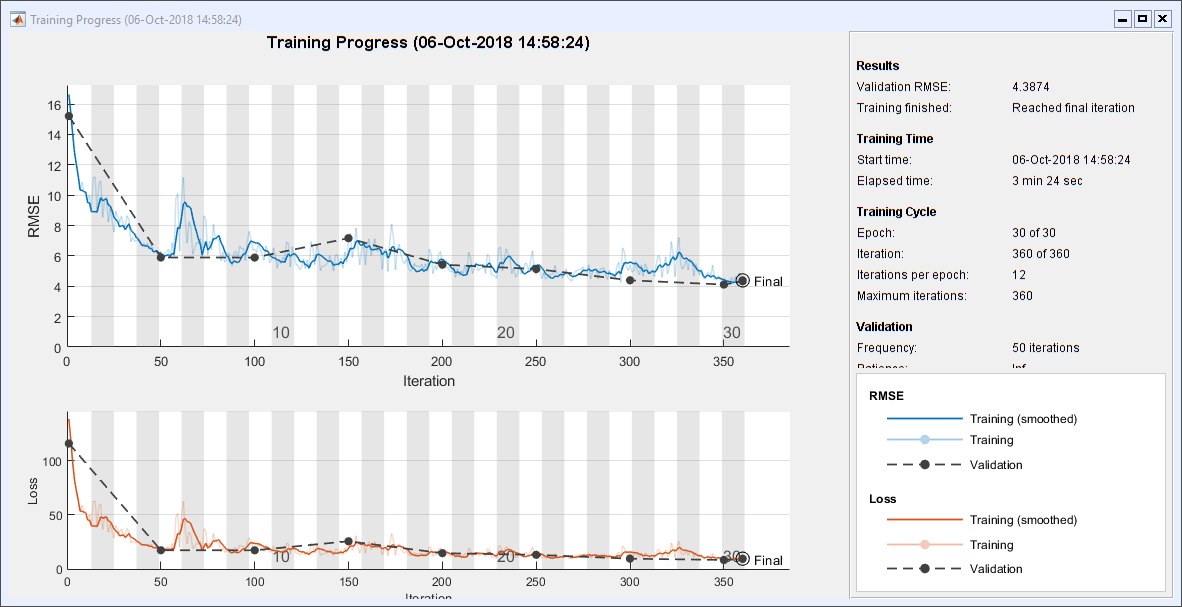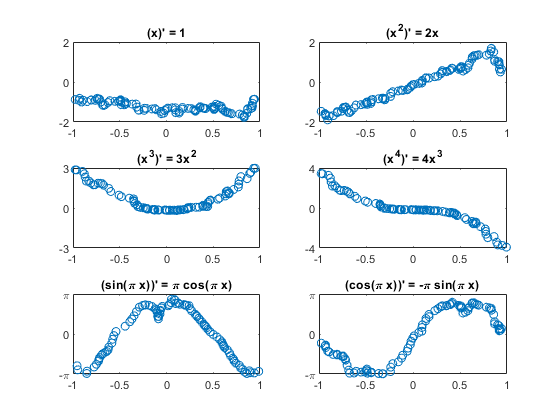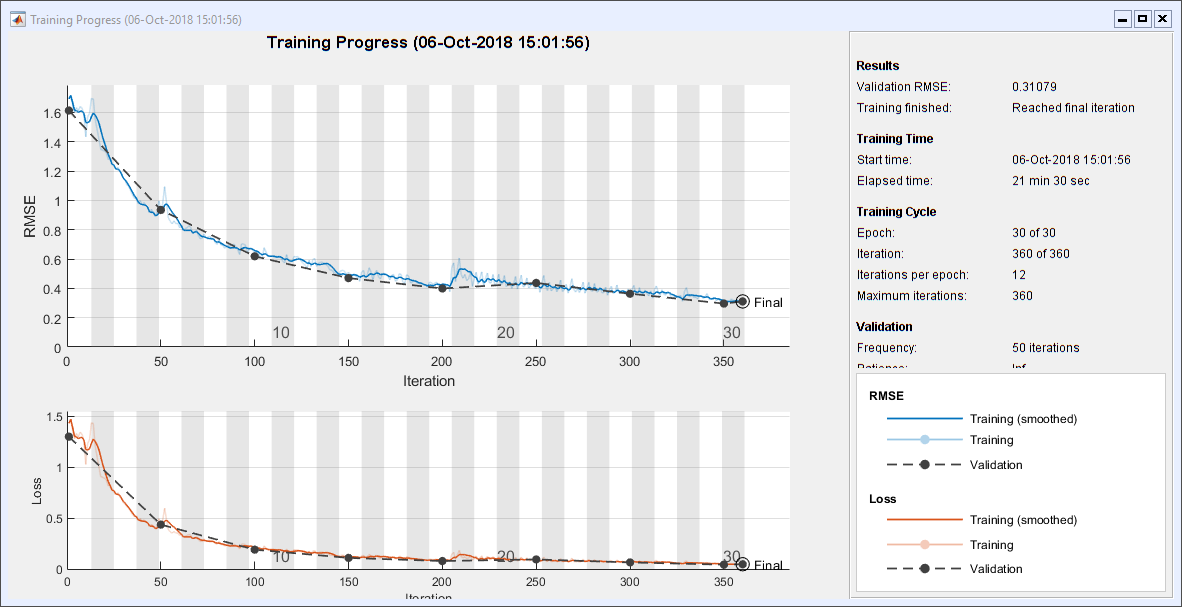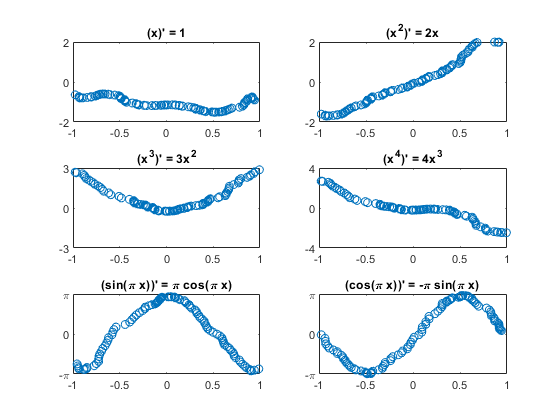# Teaching a Newcomer About Teaching Calculus to a Deep Learner3

Posted by Cleve Moler,

Two months ago I wrote a blog post about Teaching Calculus to a Deep Learner. We wrote the code for that post in one afternoon in the MathWorks booth at the SIAM Annual Meeting. Earlier that day, during his invited talk, MIT Professor Gil Strang had spontaneously wondered if it would possible to teach calculus to a deep learning computer program. None of us in the booth were experts in deep learning.

But MathWorks does have experts in deep learning. When they saw my post, they did not hesitate to suggest some significant improvements. In particular, Conor Daly, in our MathWorks UK office, contributed the code for the following post. Conor takes up the Gil's challenge and begins the process of learning about derivatives.

We are going to employ two different neural nets, a convolutional neural net, which is often used for images, and a recurrent neural net, which is often used for sounds and other signals.

Is a derivative more like an image or a sound?

### Contents

#### Functions and their derivatives

Here are the functions and derivatives that we are going to consider.

F =  {@(x) x, @(x) x.^2, @(x) x.^3, @(x) x.^4, ...
@(x) sin(pi*x), @(x) cos(pi*x) };
dF = { @(x) ones(size(x)), @(x) 2*x, @(x) 3*x.^2, @(x) 4*x.^3, ...
@(x) pi.*cos(pi.*x), @(x) -pi*sin(pi*x) };

Fchar = { 'x', 'x^2', 'x^3', 'x^4', 'sin(\pi x)', 'cos(\pi x)' };
dFchar = { '1', '2x', '3x^2', '4x^3', '\pi cos(\pi x)', '-\pi sin(\pi x)' };


#### Parameters

Set some parameters. First, the random number generator state.

rng(0)


A function to generate uniform random variables on [-1, 1].

randu = @(m,n) (2*rand(m,n)-1);


A function to generate random +1 or -1.

randsign = @() sign(randu(1,1));


The number of functions.

m = length(F);


The number of repetitions, i.e. independent observations.

n = 500;


The number of samples in the interval.

nx = 100;


The white noise level.

noise = .001;


#### Training Set

Generate the training set predictors X and the responses T.

X = cell(n*m, 1);
T = cell(n*m, 1);
for j = 1:n
x = sort(randu(1, nx));
for i = 1:m
k = i + (j-1)*m;

% Predictors are x, a random vector from -1, 1, and +/- f(x).
sgn = randsign();
X{k} = [x; sgn*F{i}(x)+noise*randn(1,nx)];

% Responses are +/- f'(x)
T{k} = sgn*dF{i}(x)+noise*randn(1,nx);
end
end


Separate the training set from the test set.

idxTest = ismember( 1:n*m, randperm(n*m, n) );
XTrain = X( ~idxTest );
TTrain = T( ~idxTest );
XTest = X( idxTest );
TTest = T( idxTest );


Choose some test indices to plot.

iTest = find( idxTest );
idxM = mod( find(idxTest), m );
idxToPlot = zeros(1, m);
for k = 0:(m-1)
im = find( idxM == k );
if k == 0
idxToPlot(m) = im(1);
else
idxToPlot(k) = im(1);
end
end


#### Convolutional Neural Network (CNN)

Re-format the data for CNN.

[XImgTrain, TImgTrain] = iConvertDataToImage(XTrain, TTrain);
[XImgTest, TImgTest] = iConvertDataToImage(XTest, TTest);


Here are the layers of the CNN architecture. Notice that the 'ReLU', or "rectified linear unit", that I was so proud of in my previous post has been replaced by the more appropriate 'leakyRelu', which does not completely cut off negative values.

layers = [ ...
imageInputLayer([1 nx 2], 'Normalization', 'none')
convolution2dLayer([1 5], 128, 'Padding', 'same')
batchNormalizationLayer()
leakyReluLayer(0.5)
convolution2dLayer([1 5], 128, 'Padding', 'same')
batchNormalizationLayer()
leakyReluLayer(0.5)
convolution2dLayer([1 5], 1, 'Padding', 'same')
regressionLayer() ];


Here are the options for CNN. The solver is 'sgdm', which stands for "stochastic gradient descent with momentum".

options = trainingOptions( ...
'sgdm', ...
'MaxEpochs', 30, ...
'Plots', 'training-progress', ...
'MiniBatchSize', 200, ...
'Verbose', false, ...
'GradientThreshold', 1, ...
'ValidationData', {XImgTest, TImgTest} );


#### Train CNN

Train the network. This requires a little over 3 minutes on my laptop. I don't have a GPU.

convNet = trainNetwork(XImgTrain, TImgTrain, layers, options);#### Plot Test Results

Here are plots of randomly selected results. The limits on the y axes are set to the theoretical max and min. Three of the six plots have their signs flipped.

PImgTest = convNet.predict( XImgTest );
for k = 1:m
subplot(3, 2, k);
plot( XImgTest(1, :, 1, idxToPlot(k)), TImgTest(1, :, 1, idxToPlot(k)), '.' )
plot( XImgTest(1, :, 1, idxToPlot(k)), PImgTest(1, :, 1, idxToPlot(k)), 'o' )
title([ '(' Fchar{k} ')'' = ' dFchar{k}  ] );
switch k
case {1,2}, set(gca,'ylim',[-2 2])
case {3,4}, set(gca,'ylim',[-k k],'ytick',[-k 0 k])
case {5,6}, set(gca,'ylim',[-pi pi],'ytick',[-pi 0 pi], ...
'yticklabels',{'-\pi' '0' '\pi'})
end
end#### Recurrent Neural Network (RNN)

Here are the layers of the RNN architecture, including 'bilstm' which stands for "bidirectional long short-term memory."

layers = [ ...
sequenceInputLayer(2)
bilstmLayer(128)
dropoutLayer()
bilstmLayer(128)
fullyConnectedLayer(1)
regressionLayer() ];


Here are the RNN options. 'adam' is not an acronym; it is an extension of stochastic gradient descent derived from adaptive moment estimation.

options = trainingOptions( ...
'adam', ...
'MaxEpochs', 30, ...
'Plots', 'training-progress', ...
'MiniBatchSize', 200, ...
'ValidationData', {XTest, TTest}, ...
'Verbose', false, ...
'GradientThreshold', 1);


#### Train RNN

Train the network. This takes almost 22 minutes on my machine. It makes me wish I had a GPU.

recNet = trainNetwork(XTrain, TTrain, layers, options);#### Plot Test Results

PTest = recNet.predict( XTest );
for k = 1:m
subplot(3, 2, k);
plot( XTest{idxToPlot(k)}(1,:), TTest{idxToPlot(k)}(1,:), '.' )
plot( XTest{idxToPlot(k)}(1,:), PTest{idxToPlot(k)}(1,:), 'o' )
title([ '(' Fchar{k} ')'' = ' dFchar{k}  ] );
switch k
case {1,2}, set(gca,'ylim',[-2 2])
case {3,4}, set(gca,'ylim',[-k k],'ytick',[-k 0 k])
case {5,6}, set(gca,'ylim',[-pi pi],'ytick',[-pi 0 pi], ...
'yticklabels',{'-\pi' '0' '\pi'})
end
end#### Convert data to CNN format

function [XImg, TImg] = iConvertDataToImage(X, T)
% Convert data to CNN format
% Re-format data for CNN
XImg = cat(4, X{:});
XImg = permute(XImg, [3 2 1 4]);
TImg = cat(4, T{:});
TImg = permute(TImg, [3 2 1 4]);
end


#### Conclusions

I used to teach calculus. I have been critical of the way calculus is sometimes taught and more often learned. Here is a typical scenario.

Instructor: What is the derivative of $x^4$?

Student: $4x^3$.

Instructor: Why?

Student: You take the $4$, put it in front, then subtract one to get $3$, and put that in place of the $4$ . . .

I am afraid we're doing that here. The learner is just looking for patterns. There is no sense of velocity, acceleration, or rate of change. The is little chance of differentiating an expression that is not in the training set. There is no product rule, no chain rule, no Fundamental Theorem of Calculus.

In short, there is little understanding. But maybe that is a criticism of machine learning in general.

Get the MATLAB code

Published with MATLAB® R2018b

### Note

Comments are closed.

## 3 CommentsOldest to Newest

Michal Kvasnicka replied on : 1 of 3
Cleve, you final statement is crucial! Any hope, that DeepLearning current state of art algorithm will be able to deeply understand anything more than simple data patterns is nearly impossible.
Cleve Moler replied on : 2 of 3
It turns out, it seems, that just recognizing simple patterns is extremely useful.
Michal Kvasnicka replied on : 3 of 3
Yes Cleve! Recognizing simple patterns is extremely useful, but only in a case of big-data. For small problems is this level of recognizing nearly trivial. I do not intend to criticize Deep Learning in general , but at present the DeepLearning is often presented as a "silver bullet" solving "every" problem. And this is definitely not true.